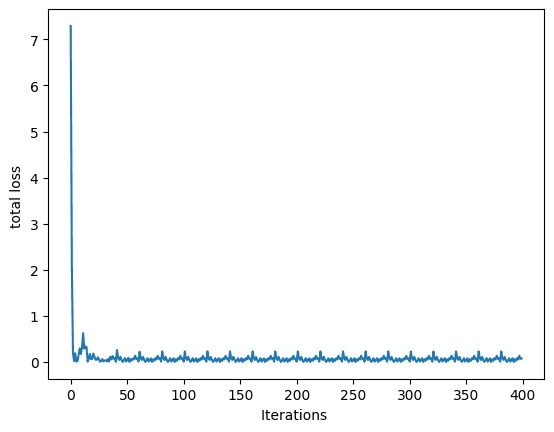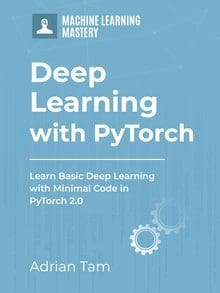# Training a Single Output Multilinear Regression Model in PyTorch

A neural network architecture is built with hundreds of neurons where each of them takes in multiple inputs to perform a multilinear regression operation for prediction. In the previous tutorials, we built a single output multilinear regression model that used only a forward function for prediction.

In this tutorial, we’ll add optimizer to our single output multilinear regression model and perform backpropagation to reduce the loss of the model. Particularly, we’ll demonstrate:

• How to build a single output multilinear regression model in PyTorch.
• How PyTorch built-in packages can be used to create complicated models.
• How to train a single output multilinear regression model with mini-batch gradient descent in PyTorch.

Kick-start your project with my book Deep Learning with PyTorch. It provides self-study tutorials with working code.

Let’s get started.Training a Single Output Multilinear Regression Model in PyTorch.
Picture by Bruno Nascimento. Some rights reserved.

## Overview

This tutorial is in three parts; they are

• Preparing Data for Prediction
• Using Linear Class for Multilinear Regression
• Visualize the Results

## Build the Dataset Class

Just like previous tutorials, we’ll create a sample dataset to perform our experiments on. Our data class includes a dataset constructor, a getter __getitem__() to fetch the data samples, and __len__() function to get the length of the created data. Here is how it looks like.

With this, we can easily create the dataset object.

### Want to Get Started With Deep Learning with PyTorch?

Take my free email crash course now (with sample code).

Click to sign-up and also get a free PDF Ebook version of the course.

## Build the Model Class

Now that we have the dataset, let’s build a custom multilinear regression model class. As discussed in the previous tutorial, we define a class and make it a subclass of nn.Module. As a result, the class inherits all the methods and attributes from the latter.

We’ll create a model object with an input size of 2 and output size of 1. Moreover, we can print out all model parameters using the method parameters().

Here’s what the output looks like.

In order to train our multilinear regression model, we also need to define the optimizer and loss criterion. We’ll employ stochastic gradient descent optimizer and mean square error loss for the model. We’ll keep the learning rate at 0.1.

## Train the Model with Mini-Batch Gradient Descent

Before we start the training process, let’s load up our data into the DataLoader and define the batch size for the training.

We’ll start the training and let the process continue for 20 epochs, using the same for-loop as in our previous tutorial.

In the training loop above, the loss is reported in each epoch. You should see the output similar to the following:

This training loop is typical in PyTorch. You will reuse it very often in future projects.

## Plot the Graph

Lastly, let’s plot the graph to visualize how the loss decreases during the training process and converge to a certain point.Loss during training

Putting everything together, the following is the complete code.

## Summary

In this tutorial you learned how to build a single output multilinear regression model in PyTorch. Particularly, you learned:

• How to build a single output multilinear regression model in PyTorch.
• How PyTorch built-in packages can be used to create complicated models.
• How to train a single output multilinear regression model with mini-batch gradient descent in PyTorch.

## Get Started on Deep Learning with PyTorch!#### Learn how to build deep learning models

...using the newly released PyTorch 2.0 library

Discover how in my new Ebook:
Deep Learning with PyTorch

It provides self-study tutorials with hundreds of working code to turn you from a novice to expert. It equips you with
tensor operation, training, evaluation, hyperparameter optimization, and much more...